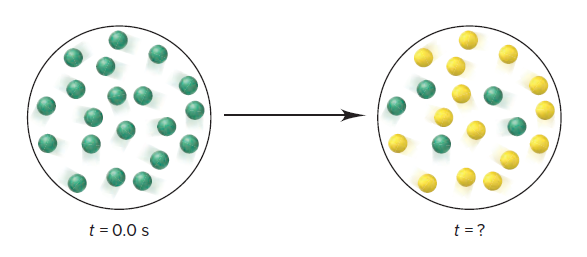# Problem: The first-order rate constant for the reaction A (green) → B (yellow) is 0.063 min-1. The scenes below represent the reaction mixture at two different times:What is the time at which the second scene occurs?

###### FREE Expert Solution
97% (446 ratings)
###### Problem Details

The first-order rate constant for the reaction A (green) → B (yellow) is 0.063 min-1. The scenes below represent the reaction mixture at two different times:What is the time at which the second scene occurs?

Frequently Asked Questions

What scientific concept do you need to know in order to solve this problem?

Our tutors have indicated that to solve this problem you will need to apply the Integrated Rate Law concept. You can view video lessons to learn Integrated Rate Law. Or if you need more Integrated Rate Law practice, you can also practice Integrated Rate Law practice problems.

What textbook is this problem found in?

Our data indicates that this problem or a close variation was asked in Chemistry: The Molecular Nature of Matter and Change - Silberberg 8th Edition. You can also practice Chemistry: The Molecular Nature of Matter and Change - Silberberg 8th Edition practice problems.